Home Practice
For learners and parents For teachers and schools
Textbooks
Full catalogue
Pricing SupportLog in

We think you are located in United States. Is this correct?

# Calculations using whole numbers

## 1.4 Calculations using whole numbers

### Estimation

To estimate is to try to get close to an answer without actually doing the required calculations with the given numbers. An estimate may also be called an approximation. The difference between an estimate and the actual answer is called the error.

Calculating with “easy” numbers that are close to given numbers is a good way to obtain approximate answers (or estimates), for example:

• To approximate $$764 + 829$$, you could calculate $$800 + 800$$ to get the approximate answer $$1\ 600$$, with an error of $$7$$.
• To approximate $$84 \times 178$$, you could calculate $$80 \times 200$$ to get the approximate answer $$16\ 000$$, with an error of $$1\ 048$$.

## Worked Example 1.6: Finding approximate answers

Calculate with “easy” numbers close to the given numbers to produce approximate answers for this product. Do not use a calculator. When you have made your approximations, use a calculator to find the exact answer and calculate the error.

$78 \times 46$

### Find easy numbers close to the given numbers.

$80 \times 40$

You could also take $$80$$ and $$50$$ as your easy numbers.

### Multiply without using a calculator.

$80 \times 40 = 3\ 200$

### Use a calculator to find the actual answer.

$78 \times 46 = 3\ 588$

### Find the error in calculation.

The actual answer is bigger than the approximation.

$3\ 588 - 3\ 200 = 388$

The error is $$388$$, so the estimation was an underestimation.

## Worked Example 1.7: Finding approximate answers

Calculate using easy numbers, and without using a calculator. When you have made your approximations, use a calculator to find the precise answer and calculate the error.

$78 \times 178$

### Find easy numbers close to the given numbers.

$80 \times 200$

### Multiply.

$80 \times 200 = 16\ 000$

### Use a calculator to find the actual answer.

$78 \times 178 = 13\ 884$

### Find the error in calculation.

The actual answer is smaller than the approximation.

$16\ 000 - 13\ 884 = 2\ 116$

The error is $$2\ 116$$, so the estimation was an overestimation.

This is quite a large error. Imagine that you were cutting wood to make furniture and your error was $$2\ 116 \text{ mm}$$. If each piece of wood was $$2\ 116 \text{ mm}$$ wider than it should be, you would have a problem making your furniture!

Another two easy numbers you could have chosen are $$70 \times 200 = 14\ 000$$. The second number is quite far from $$200$$, so it is better to choose a lower first number. The error in this calculation is then much lower: $$14\ 000 - 13\ 884 = 116$$.

Exercise 1.1: Approximate the answers to large products

Calculate with easy numbers close to the given numbers to produce approximate answers for each product below. Do not use a calculator. When you have made your approximations, use a calculator to find the precise answers.

1. $88 \times 56$
2. $77 \times 98$
3. $94 \times 275$
4. $71 \times 198$
5. $1\ 250 \times 79$
1. $88 \times 56$

Approximation: $$90 \times 50 = 4\ 500$$

Actual answer: $$4\ 928$$

2. $77 \times 98$

Approximation: $$80 \times 100 = 8\ 000$$

Actual answer: $$7\ 546$$

3. $94 \times 275$

Approximation: $$90 \times 300 = 27\ 000$$

Actual answer: $$25\ 850$$

4. $71 \times 198$

Approximation: $$70 \times 200 = 14\ 000$$

Actual answer: $$14\ 058$$

5. $1\ 250 \times 79$

Approximation: $$1\ 000 \times 80 = 80\ 000$$

Actual answer: $$98\ 750$$

### Methods for adding, subtracting and multiplying

Let’s revise some basic addition, subtraction and multiplication facts.

• Remember that the sum is what you get after you have done your addition. We can add in any order.

$44 + 41 = 85$

The sum of $$44$$ and $$41$$ is $$85$$.

• The difference is what is left after you have done your subtraction. We must subtract in the given order.

$55 - 15 = 40$

The difference between $$55$$ and $$15$$ is $$40$$.

• There are lots of ways to subtract numbers from each other. This regrouping method is just one example.

$(55 - 5) - 10 = 50 - 10 = 40$
• The product is what you get after you have done your multiplication. We can multiply in any order.

$5 \times 10 = 50$

The product of $$5$$ and $$10$$ is $$50$$.

• The quotient is what is left after you have done your division. We must divide in the given order.

To determine the quotient when $$30$$ is divided by $$5$$, we must divide $$30$$ by $$5$$.

$30 \div 5 = 6$

The quotient is $$6$$.

temp text

Numbers can be added by thinking of their parts as we say the numbers. For example, we say $$4\ 994$$ as ‘four thousand nine hundred and ninety-four’. This can be written in expanded notation as $$4\ 000 + 900 + 90 + 4$$.

Similarly, we can think of $$31\ 837$$ as $$30\ 000 + 10\ 000 + 800 + 30 + 7$$.

$$31\ 837 + 4\ 994$$ can be calculated by working with the various kinds of parts separately. To make this easy, the numbers can be written below each other so that the units are below the units, the tens below the tens and so on, as shown here.

$\begin{array}{ccccc} 3 & 1 & 8 & 3 & 7 \\ & 4 & 9 & 9 & 4 \end{array}$

Always align the numbers starting from ones and moving to the bigger units, in other words, from right to left.

We can now expand each number:

$\begin{array}{rccccc} \mathbf{31\ 837} & 30\ 000 & 1\ 000 & 800 & 30 & 7 \\ \mathbf{4\ 994} & & 4\ 000 & 900 & 90 & 4 \end{array}$

Add the numbers in each column separately, to get a new set of numbers that you can write at the far left.

$\begin{array}{rccccc} \mathbf{31\ 837} & 30\ 000 & 1\ 000 & 800 & 30 & 7 \\ \mathbf{4\ 994} & & 4\ 000 & 900 & 90 & 4 \\ \hline 11 & & & & & 11 \\ 120 & & & & 120 & \\ 1\ 700 & & & 1\ 700 & & \\ 5\ 000 & & 5\ 000 & & & \\ 30\ 000 & 30\ 000 & & & & \\ \hline 36\ 831 & & & & & \end{array}$

It is easy to add the new set of numbers to get the answer:

$30\ 000 + 5\ 000 + 1\ 700 + 120 + 11 = 36\ 831$

Starting with the ones as shown above makes it possible to do more of the work mentally, and write less, as shown below.

$\begin{array}{ccccc} & 3 & ^{1}1 & ^{1}8 & ^{1}3 & 7 \\ & & 4 & 9 & 9 & 4 \\ \hline & 3 & 6 & 8 & 3 & 1 \end{array}$

To achieve this, the first step is to add the digits in the ones column: $$7 + 4 = 11$$. Only write the digit “$$1$$” of the $$11$$. The $$10$$ of the $$11$$ is remembered and added to the $$3$$ in the tens column.

We say the $$10$$ is carried from the ones column to the tens column. The same is done when the tens parts are added to get $$13$$: only the digit “$$3$$” is written (in the tens column, so it means $$30$$), and the $$1$$ is carried to the next column. This $$1$$ is actually $$100$$, so it needs to be carried to the hundreds column.

## Worked Example 1.8: Adding whole numbers

Calculate without using a calculator:

$4\ 638 + 2\ 667$

### Break the two numbers into parts.

\begin{align} 4\ 638 &= 4\ 000 + 600 + 30 + 8 \\ 2\ 667 &= 2\ 000 + 600 + 60 + 7 \end{align}

### Write the numbers below each other using place value, and add up the numbers in columns.

$\begin{array}{rcccc} \mathbf{4\ 638} & 4\ 000 & 600 & 30 & 8 \\ \mathbf{2\ 667} & 2\ 000 & 600 & 60 & 7 \\ \hline \mathbf{15} & & & & 15 \\ \mathbf{90} & & & 90 & \\ \mathbf{1\ 200} & & 1\ 200 & & \\ \mathbf{6\ 000} & 6\ 000 & & & \\ \hline \mathbf{7\ 305} & & & & \end{array}$

$6\ 000 + 1\ 200 + 90 + 15 = 7\ 305$

## Worked Example 1.9: Adding whole numbers

Calculate without using a calculator:

$10\ 748 + 7\ 246$

### Break the two numbers into parts.

\begin{align} 10\ 748 = 10\ 000 + 700 + 40 + 8 \\ 7\ 246 = 7\ 000 + 200 + 40 + 6 \end{align}

### Write the numbers below each other and add up the numbers in columns.

$\begin{array}{rcccc} \mathbf{10\ 748} & 10\ 000 & 700 & 40 & 8 \\ \mathbf{7\ 246} & 7\ 000 & 200 & 40 & 6 \\ \hline \mathbf{14} & & & & 14 \\ \mathbf{80} & & & 80 & \\ \mathbf{900} & & 900 & & \\ \mathbf{17\ 000} & 17\ 000 & & & \\ \hline \mathbf{17\ 994} & & & & \end{array}$

$17\ 000 + 900 + 80 + 14 = 17\ 994$

## Worked Example 1.10: Adding whole numbers

Impilo Enterprises plans a new computerised training facility in their existing building. The training manager has to keep the total expenditure budget under $$\text{R } 1 \text{ million}$$. This is what she has written so far.

 Architects and builders $$\text{R} 102\ 700$$ Painting and carpeting $$\text{R} 42\ 600$$ Security doors and blinds $$\text{R} 52\ 000$$ Data projector $$\text{R } 4\ 800$$ $$25$$ new secretary chairs $$\text{R} 50\ 400$$ $$24$$ desks for work stations $$\text{R} 123\ 000$$ $$1$$ desk for presenter $$\text{R} 28\ 000$$ $$25$$ new computers $$\text{R} 300\ 000$$ $$12$$ colour laser printers $$\text{R} 38\ 980$$

Work out the total cost of all the items the training manager has budgeted for.

### Break each number into parts (expand the numbers).

 Architects and builders $$\text{R} 102\ 700$$ $$102\ 700 = 100\ 000 + 2\ 000 + 700$$ Painting and carpeting $$\text{R} 42\ 600$$ $$42\ 600 = 40\ 000 + 2\ 000 + 600$$ Security doors and blinds $$\text{R} 52\ 000$$ $$52\ 000 = 50\ 000 + 2\ 000$$ Data projector $$\text{R} 4\ 800$$ $$4\ 800 = 4\ 000 + 800$$ $$25$$ new secretary chairs $$\text{R} 50\ 400$$ $$50\ 400 = 50\ 000 + 400$$ $$24$$ desks for work stations $$\text{R} 123\ 000$$ $$123\ 000 = 100\ 000 + 20\ 000 + 3\ 000$$ $$1$$ desk for presenter $$\text{R} 28\ 000$$ $$28\ 000 = 20\ 000 + 8\ 000$$ $$25$$ new computers $$\text{R} 300\ 000$$ $$300\ 000 = 300\ 000$$ $$12$$ colour laser printers $$\text{R} 38\ 980$$ $$38\ 980 = 30\ 000 + 8\ 000 + 900 + 80$$

### Write the numbers below each other and add up the numbers in columns.

Be careful as you place the numbers according to their place value!

$\begin{array}{rccccc} \mathbf{102\ 700} & 100\ 000 & & 2\ 000 & 700 & \\ \mathbf{42\ 600} & & 40\ 000 & 2\ 000 & 600 & \\ \mathbf{52\ 000} & & 50\ 000 & 2\ 000 & & \\ \mathbf{4\ 800} & & & 4\ 000 & 800 & \\ \mathbf{50\ 400} & & 50\ 000 & & 400 & \\ \mathbf{123\ 000} & 100\ 000 & 20\ 000 & 3\ 000 & & \\ \mathbf{28\ 000} & & 20\ 000 & 8\ 000 & & \\ \mathbf{300\ 000} & 300\ 000 & & & & \\ \mathbf{38\ 980} & & 30\ 000 & 8\ 000 & 900 & 80 \\ \hline & \mathbf{500\ 000} & \mathbf{210\ 000} & \mathbf{29\ 000} & \mathbf{3\ 400} & \mathbf{80} \end{array}$

When there are many numbers, it is easier to write the sums of place values in one row.

\begin{align} & 500\ 000 + 210\ 000 + 29\ 000 + 3\ 400 + 80 \\ &= 710\ 000 + 32\ 480 \\ &= 742\ 480 \end{align}
temp text

### Methods of subtraction

There are many ways to find the difference between two numbers. For example, to find the difference between $$267$$ and $$859$$, you can think of the numbers as they may be written on a number line.

We may think of the distance between $$267$$ and $$859$$ as three steps: from $$267$$ to $$300$$, from $$300$$ to $$800$$, and from $$800$$ to $$859$$. How big are each of these three steps?

This number line shows that $$859 - 267$$ is $$33 + 500 + 59$$. This means that $$859 - 267 = 592$$.

Like addition, subtraction can also be done by working with the different parts in which we say (or expand) the numbers. For example, $$8\ 764 − 2\ 352$$ can be calculated as follows:

• $$8$$ thousand − $$2$$ thousand = $$6$$ thousand
• $$7$$ hundred − $$3$$ hundred = $$4$$ hundred
• $$6$$ tens − $$5$$ tens = $$1$$ ten
• $$4$$ units − $$2$$ units = $$2$$ units

So, $$8\ 764 − 2\ 352 = 6\ 412$$.

Subtraction by parts is more difficult in some cases, for example $$6\ 213 − 2\ 758$$:

$$6\ 000 - 2\ 000 = 4\ 000$$ This step is easy, but the following steps cause problems:

• $$200 - 700 = \ ?$$
• $$10 - 50 = \ ?$$
• $$3 - 8 = \ ?$$

One way to overcome these problems is to work with negative numbers:

• $$200 - 700 = - 500$$
• $$10 - 50 = - 40$$
• $$3 - 8 = - 5$$

\begin{align} 6\ 213 - 2\ 758 &= 4\ 000 + ( - 500) + ( - 40) + ( - 5) \\ &= 3\ 500 + ( - 45) \\ &= 3\ 455 \end{align}

In this method, it is easy to make mistakes if you are not careful with the minus sign. Fortunately, the parts and sequence of work may be rearranged to overcome these problems, as shown below. We regroup both numbers to make subtraction in columns easier:

\begin{align} 6\ 213 &= 6\ 000 + 200 + 10 + 3 \\ &= 5\ 000 + 1\ 100 + 100 + 13 \end{align}
 $$\mathbf{+ 1\ 000}$$ (borrow $$1\ 000$$) $$\mathbf{+ 100}$$ (borrow $$100$$) $$\mathbf{+ 10}$$ (borrow $$10$$) $$1^{\text{st}}$$ number: $$6\ 213$$ $$6\ 000 = 5\ 000 + 1\ 000$$ $$200 = 100 + 100$$ $$10$$ $$3$$ $$5\ 000$$ $$1\ 100$$ $$100$$ $$13$$ $$2^{\text{nd}}$$ number: $$2\ 758$$ $$2\ 000$$ $$700$$ $$50$$ $$8$$ $$5\ 000 − 2\ 000 = 3\ 000$$ $$1\ 100 − 700 = 400$$ $$100 − 50 = 50$$ $$13 − 8 = 5$$

Now it is easier to find the answer: $$3\ 000 + 400 + 50 + 5 = 3\ 455$$.

This reasoning can also be set out in columns:

With some practice, you can learn to subtract using borrowing without writing all the steps. It is convenient to work in columns.

## Worked Example 1.11: Subtracting whole numbers

Calculate: $$7\ 342 − 3\ 877$$

### Write the two numbers in place value columns.

 $$7$$ $$3$$ $$4$$ $$2$$ $$−$$ $$3$$ $$8$$ $$7$$ $$7$$

### Check where the top number is smaller than the bottom number, and exchange bigger units for smaller units, from right to left:

 $$7^{−1}$$ $$^{+1}3^{−1}$$ $$^{+1}4^{−1}$$ $$^{+1}2$$ $$−$$ $$3$$ $$8$$ $$7$$ $$7$$ $$1\ 000$$ went to the hundreds column, so $$6\ 000$$ is left. $$1$$ hundred went to the tens column. $$200 < 800$$, so get $$1\ 000$$ units from the left column. $$1$$ ten went to the units column. $$30 < 70$$, so get $$100$$ units from the left column $$2 < 7$$, so get $$10$$ units from the left column.

### Subtract inside each place value column to get the final answer:

 $$7^{−1}$$ $$^{+1}3^{−1}$$ $$^{+1}4^{−1}$$ $$^{+1}2$$ $$−$$ $$3$$ $$8$$ $$7$$ $$7$$ $$6 − 3 = 3$$ $$12 − 8 = 4$$ $$13 − 7 = 6$$ $$12 − 7 = 5$$

The answer is $$7\ 342 − 3\ 877 = 3\ 465$$.

## Worked Example 1.12: Subtracting whole numbers

Calculate: $$8\ 653 − 1\ 856$$

### Write the two numbers in place value columns.

 $$8$$ $$6$$ $$5$$ $$3$$ $$−$$ $$1$$ $$8$$ $$5$$ $$6$$

### Check where the top number is smaller than the bottom number, and exchange bigger units for smaller units, from right to left.

 $$8^{−1}$$ $$^{+1}6^{−1}$$ $$^{+1}5^{−1}$$ $$^{+1}3$$ $$−$$ $$1$$ $$8$$ $$5$$ $$6$$ $$1\ 000$$ went to the hundreds column, so $$7\ 000$$ is left $$1$$ hundred went to tens column. $$500 < 800$$, so get $$1\ 000$$ units from the left column $$1$$ ten went to units column. $$40 < 50$$, so get $$100$$ units from the left column $$3 < 6$$, so get $$10$$ units from the left column

### Subtract inside each place value column to get the final answer.

 $$8^{−1}$$ $$^{+1}6^{−1}$$ $$^{+1}5^{−1}$$ $$^{+1}3$$ $$−$$ $$1$$ $$8$$ $$5$$ $$6$$ $$7 − 1 = 6$$ $$15 − 8 = 7$$ $$14 − 5 = 9$$ $$13 − 6 = 7$$

The answer is $$8\ 653 − 1\ 856 = 6\ 797$$.

Exercise 1.2: Subtraction with large numbers

Calculate each of the following

1. $4\ 340 - 3\ 870$
2. $1\ 653 - 1\ 156$
3. $3\ 671 - 2\ 528$
1. $4\ 340 - 3\ 870 = 470$
2. $1\ 653 - 1\ 156 = 497$
3. $3\ 671 - 2\ 528 = 1\ 143$

Estimate the difference between the two car prices in each case to the nearest $$\text{R } 1\ 000$$ or closer. Then calculate the difference.

1. $$\text{R } 102\ 365$$ and $$\text{R } 98\ 128$$
2. $$\text{R } 63\ 378$$ and $$\text{R } 96\ 889$$
1. $$\text{R } 102\ 365$$ and $$\text{R } 98\ 128$$

Estimate: $$\text{R } 102\ 000 − \text{R } 98\ 000 = \text{R } 4\ 000$$

Actual: $$\text{R } 4\ 237$$

2. $$\text{R } 63\ 378$$ and $$\text{R } 96\ 889$$

Estimate: $$\text{R } 97\ 000 − \text{R } 63\ 000 = \text{R } 34\ 000$$

Actual: $$\text{R } 33\ 511$$

• First estimate the answers to the nearest $$100\ 000$$ or $$10\ 000$$ or $$1\ 000$$. Then calculate.
1. $238\ 769 - 141\ 453$
2. $856\ 333 - 739\ 878$
3. $65\ 244 - 39\ 427$
1. $238\ 769 - 141\ 453$

Estimate: $$240\ 000 − 140\ 000 = 100\ 000$$

Actual: $$97\ 316$$

2. $856\ 333 - 739\ 878$

Estimate: $$860\ 000 − 740\ 000 = 120\ 000$$

Actual: $$116\ 455$$

3. $65\ 244 - 39\ 427$

Estimate: $$65\ 000 − 40\ 000 = 25\ 000$$

Actual: $$25\ 817$$

### A method of multiplication

Just as with addition and subtraction, we can use the method of breaking up numbers into parts. For example, to find the product $$7 \times 4\ 598$$, we can expand $$4\ 596$$ like this:

• $$7 \times 4\ 000 = 28\ 000$$
• $$7 \times 500 = 3\ 500$$
• $$7 \times 90 = 630$$
• $$7 \times 8 = 56$$

The four partial products can now be added to get the answer, which is $$32\ 186$$. It is convenient to write the work in vertical columns for units, tens, hundreds and so on, as shown below.

$\begin{array}{rccccc} & & 4 & 5 & 9 & 8 \\ \times & & & & & 7 \\ \hline & & & & 5 & 6 \\ & & & 6 & 3 & 0 \\ & & ^{1}3 & 5 & 0 & 0 \\ + & ^{1}2 & 8 & 0 & 0 & 0 \\ \hline & 3 & 2 & 1 & 8 & 6 \end{array}$

The answer can be produced with less writing by “carrying” parts of the partial answers to the next column, when working from right to left in the columns.

$\begin{array}{rccccc} & & ^{4}4 & ^{6}5 & ^{5}9 & 8 \\ \times & & & & & 7 \\ \hline & 3 & 2 & 1 & 8 & 6 \end{array}$

Only the 6 of the product $$7 \times 8$$ is written down instead of $$56$$. The $$50$$ is kept in mind, and added to the $$630$$ obtained when $$7 \times 90$$ is calculated in the next step.

## Worked Example 1.13: Multiplying whole numbers

Calculate each of the following. Do not use a calculator.

$27 \times 649$

### Write the two numbers in columns, start with the bigger number.

$\begin{array}{rccc} & 6 & 4 & 9 \\ \times & & 2 & 7 \end{array}$

### Multiply by the units of the second number first.

$\begin{array}{rcccc} & & ^{3}6 & ^{6}4 & 9 \\ \times & & & 2 & 7 \\ \hline & 4 & 5 & 4 & 3 \end{array}$

### Multiply by the tens of the second number. Start writing in the tens column.

$\begin{array}{rccccc} & & & 6 & ^{1}4 & 9 \\ \times & & & & 2 & 7 \\ \hline & & 4 & 5 & 4 & 3 \\ & 1 & 2 & 9 & 8 & \end{array}$

$\begin{array}{rccccc} & & & ^{3}6 & ^{6}4 & 9 \\ \times & & & & 2 & 7 \\ \hline & & ^{1}4 & ^{1}5 & 4 & 3 \\ + & 1 & 2 & 9 & 8 & \\ \hline & 1 & 7 & 5 & 2 & 3 \end{array}$

The answer is $$27 \times 649 = 17\ 523$$.

## Worked Example 1.14: Multiplying whole numbers

Calculate without using a calculator:

$3\ 487 \times 93$

### Write the two numbers in column, start with the bigger number.

$\begin{array}{rcccc} & 3 & 4 & 8 & 7 \\ \times & & & 9 & 3 \end{array}$

### Multiply by the units of the second number first.

$\begin{array}{rccccc} & & ^{1}3 & ^{2}4 & ^{2}8 & 7 \\ \times & & & & 9 & 3 \\ \hline & 1 & 0 & 4 & 6 & 1 \end{array}$

### Multiply by the tens of the second number.

$\begin{array}{rcccccc} & & & ^{4}3 & ^{7}4 & ^{6}8 & 7 \\ \times & & & & & 9 & 3 \\ \hline & & 1 & 0 & 4 & 6 & 1 \\ & 3 & 1 & 3 & 8 & 3 & \end{array}$

$\begin{array}{rcccccc} & & & 3 & 4 & 8 & 7 \\ \times & & & & & 9 & 3 \\ \hline & & 1 & ^{1}0 & 4 & 6 & 1 \\ + & 3 & 1 & 3 & 8 & 3 & \\ \hline & 3 & 2 & 4 & 2 & 9 & 1 \end{array}$

The answer is $$3\ 487 \times 93 = 324\ 291$$.

Exercise 1.3

Calculate each of the following. Do not use a calculator now.

1. $29 \times 549$
2. $78 \times 1\ 750$
3. $641 \times 99$

1. $29 \times 549 = 15\ 921$
2. $78 \times 1\ 750 = 136\ 500$
3. $641 \times 99 = 63\ 459$

Calculate each of the following. Do not use a calculator now.

1. $97 \times 176$
2. $74 \times 197$
1. $97 \times 176 = 17\ 072$
2. $74 \times 197 = 14\ 578$

### Long division method

Long division is a useful method for dividing large numbers without a calculator. Essentially, you break the division problem into lots of similar steps, and follow a sequence.

## Worked Example 1.15: Dividing whole numbers

Use long division method to find the answer to $$9\ 396 \div 27$$.

### Set up the calculation in columns.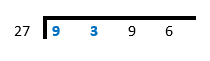### Divide.

Ask the question: “How many times does $$27$$ go into $$93$$?”

You choose $$93$$ because $$27$$ cannot go into $$9$$, the very first digit.

The answer is “$$3$$ times”, because $$27 \times 3 = 81$$. You write $$3$$ above the division line and $$81$$ below $$93$$.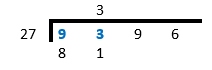### Subtract and bring the next digit down.

$93 - 81 = 12$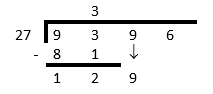### Divide again (repeat Step 2).

Ask the question: “How many times does $$27$$ go into $$129$$?”

The answer is “$$4$$ times”, because $$27 \times 4 = 108$$. You write $$4$$ above the division line and $$108$$ below $$129$$.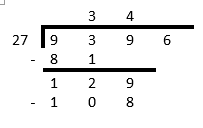### Subtract and bring the next digit down (repeat Step 3).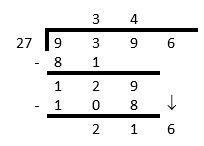### Divide again (repeat Step 2).

Ask the question: “How many times does $$27$$ go into $$216$$?”

The answer is “$$8$$ times”, because $$27 \times 8 = 216$$. You write $$8$$ above the division line and $$216$$ below $$216$$.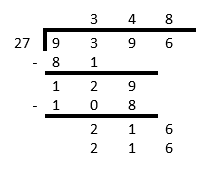### Subtract.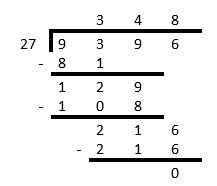We now stop this calculation. The remainder of the division is $$0$$.

The answer is $$9\ 396 \div 27 = 348$$.

## Worked Example 1.16: Dividing whole numbers

Use long division to calculate $$4\ 125 \div 12$$.

### Set up the calculation in columns.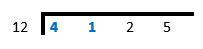### Divide.

Ask the question: “How many times does $$12$$ go into $$41$$?”

You choose $$41$$ because $$12$$ cannot go into $$4$$, the very first digit.

The answer is “$$3$$ times”, because $$12 \times 3 = 36$$. You write $$3$$ above the division line and $$36$$ below $$41$$.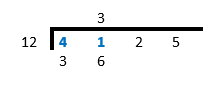### Subtract and bring the next digit down.

$41 - 36 = 5$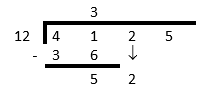### Divide again (repeat Step 2).

Ask the question: “How many times does $$12$$ go into $$52$$?”

The answer is “$$4$$ times”, because $$12 \times 4 = 48$$. You write $$4$$ above the division line and $$48$$ below $$52$$.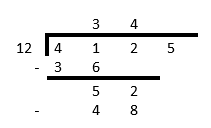### Subtract and bring the next digit down (repeat Step 3).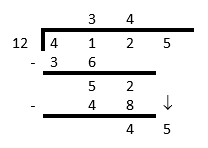### Divide again (repeat Step 2).

Ask the question: “How many times does $$12$$ go into $$45$$?”

The answer is “$$3$$ times”, because $$12 \times 3 = 36$$. You write $$3$$ above the division line and $$36$$ below $$45$$.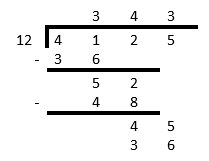### Subtract.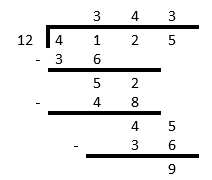We now stop this calculation. The remainder of the division is $$9$$.

So, the answer is $$4\ 125 \div 12 = 343$$ with a remainder of $$9$$.

Check: $$343 \times 12 + 9 = 4 116 + 9 = 4\ 125$$.

Exercise 1.4: Calculations with division

Brenda bought $$64$$ goats, all at the same price. She paid $$\text{R} 5\ 440$$ in total. What was the price for each goat?

Your first step can be to work out how much Brenda would have paid if she paid $$\text{R} 10$$ per goat, but you can start with a bigger step if you wish.

$5\ 440 \div 64 = \text{R}85$

Mosibudi has $$\text{R} 2\ 850$$ and he wants to buy candles for his sister’s wedding reception. The candles cost $$\text{R} 48$$ each. How many candles can he buy?

$$2\ 850 \div 48 = 59 \text{ rem } 18$$, so he can buy $$59$$ candles.

Calculate each of the following, without using a calculator.

1. $7\ 234 \div 48$
2. $3\ 267 \div 24$
3. $9\ 500 \div 364$
4. $8\ 347 \div 24$
1. $7\ 234 \div 48 = 150 \text{ rem } 34$
2. $3\ 267 \div 24 = 136 \text{ rem } 3$
3. $9\ 500 \div 364 = 26 \text{ rem } 36$
4. $8\ 347 \div 24 = 347 \text{ rem } 19$